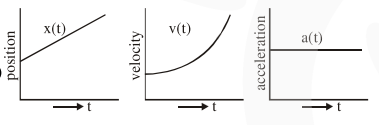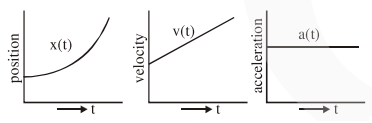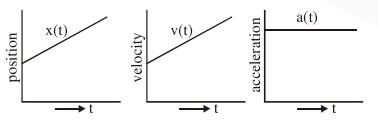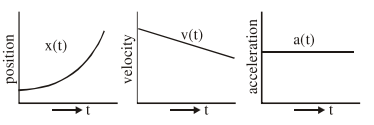# The position, velocity and acceleration of a particleQuestion:

The position, velocity and acceleration of a particle moving with a constant acceleration can be represented by :

1.2.3.4.Correct Option: , 2

Solution:

Option (2) represent correct graph for particle moving with constant acceleration, as for constant acceleration velocity time graph is straight line with positive slope and $x$-t graph should be an opening upward parabola.